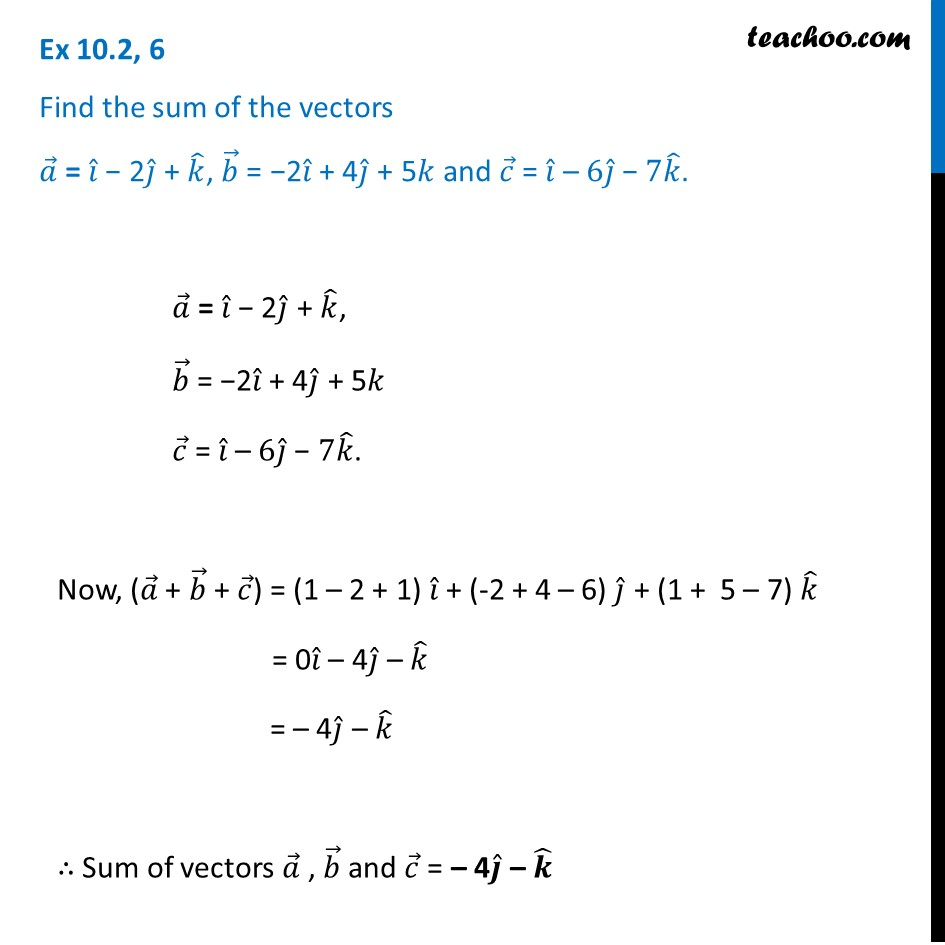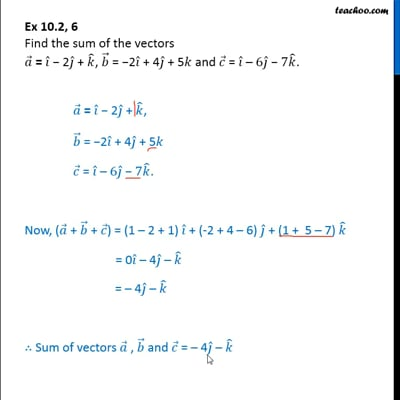Chapter 10 Class 12 Vector Algebra
Concept wiseThis video is only available for Teachoo black users

Introducing your new favourite teacher - Teachoo Black, at only ₹83 per month

### Transcript

Ex 10.2, 6 Find the sum of the vectors 𝑎 ⃗ = 𝑖 ̂ − 2𝑗 ̂ + 𝑘 ̂, 𝑏 ⃗ = −2𝑖 ̂ + 4𝑗 ̂ + 5𝑘 and 𝑐 ⃗ = 𝑖 ̂ – 6𝑗 ̂ − 7𝑘 ̂. 𝑎 ⃗ = 𝑖 ̂ − 2𝑗 ̂ + 𝑘 ̂, 𝑏 ⃗ = −2𝑖 ̂ + 4𝑗 ̂ + 5𝑘 𝑐 ⃗ = 𝑖 ̂ – 6𝑗 ̂ − 7𝑘 ̂. Now, (𝑎 ⃗ + 𝑏 ⃗ + 𝑐 ⃗) = (1 – 2 + 1) 𝑖 ̂ + (-2 + 4 – 6) 𝑗 ̂ + (1 + 5 – 7) 𝑘 ̂ = 0𝑖 ̂ – 4𝑗 ̂ – 𝑘 ̂ = – 4𝑗 ̂ – 𝑘 ̂ ∴ Sum of vectors 𝑎 ⃗ , 𝑏 ⃗ and 𝑐 ⃗ = – 4𝒋 ̂ – 𝒌 ̂